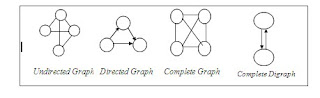/* related posts with thumb nails */

###Graphs & types of graphs.:

4 A graph is a collection of vertices or nodes, which are joined as pairs by lines or edges.

4 Formally, a graph G = (V, E) is an ordered pair of finite sets of Vertices and Edges.

4 Vertices are also called as nodes or points

4 Edges are called as lines or arcs.

4 Vertices are displayed as circles and edges are displayed as lines.

4 An edge with orientation (à) is directed edge while an edge with no orientation (¾) is undirected edge.The types of graphs are listed below:

1. Directed Graph: A graph in which each edge is directed is called Directed graph.

2. Undirected graph: A graph in which each edge is undirected is called an undirected graph.

3. Connected graph: A graph G is connected if there is a path between every pair of vertices.

4. Sub graph: sub graph is a graph in which vertex and edge sets are subsets of those of G.

5. Complete Graph: A graph G is said to be complete if every vertex V is adjacent to every other vertex V in G. A complete graph with ‘n’ vertices has ‘n (n-1)/2’ edges.

6. Weighted graph: A graph ‘G’ is weighted, if each edge in G is assigned a nonnegative numerical value (cost or weight).

7. Connected directed graph or strongly connected graph: A directed graph G is said to be connected, or strongly connected, if for each pair of vertices (v1, v2) there is a path from v1 to v2 and v2 to v1.

Related Topics: